Courses

# Quant Mock Test - 8

## 20 Questions MCQ Test Mock Test Series for CLAT 2020 | Quant Mock Test - 8

Description
This mock test of Quant Mock Test - 8 for CLAT helps you for every CLAT entrance exam. This contains 20 Multiple Choice Questions for CLAT Quant Mock Test - 8 (mcq) to study with solutions a complete question bank. The solved questions answers in this Quant Mock Test - 8 quiz give you a good mix of easy questions and tough questions. CLAT students definitely take this Quant Mock Test - 8 exercise for a better result in the exam. You can find other Quant Mock Test - 8 extra questions, long questions & short questions for CLAT on EduRev as well by searching above.
QUESTION: 1

### If sum of two numbers be a and their product be b, then the sum of their reciprocals is

Solution:

If the number be x and y, then
x + y = a and xy = b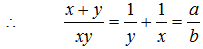QUESTION: 2

### In a camp of 160 students provisions are available for 10 days. If 40 more students join the camp, how long will be provision last?

Solution:

By, M1D1 = M2D2
⇒160 x 10 = 200 x D2
⇒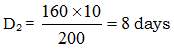QUESTION: 3

### Discount on a pair of shoes marked at Rs. 475 and discounted at 15%, is

Solution:

Required discount =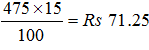QUESTION: 4

There taps A, B, C can fill an overhead tank in 4, 6 and 12 hours respectively. How long would be three taps take to fill the tank if all of them are opened together?

Solution:

Part of the tank filled by all three taps in an hour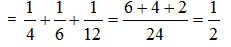∴ Hence, the tank will be filled in 2 hours.

QUESTION: 5

The cost price of an article is Rs. 100. A discount series of 5%, 10% successively reduces the price of a article by

Solution:

Single equivalent discount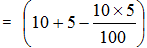= 14.5%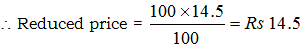QUESTION: 6

A grinder was marked at Rs. 3,600. After given a discount of 10% the dealer made a profit of 8%. Calculate the cost price.

Solution:

Let the C.P. of grinder be = Rs x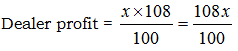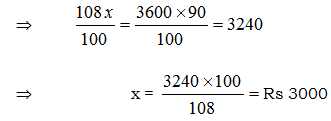QUESTION: 7

If x2 + 9y2 = 6xy, then x : y is

Solution:

x2 + 9y2 = 6xy

⇒ x2 – 6xy + 9y2 = 0

⇒ x2 – 2x.3y + (3y)2 = 0

⇒ (x – 3y)2 = 0

⇒ x – 3y = 0

⇒ x = 3y

⇒ x : y = 3 : 1

QUESTION: 8

The average of 8 numbers is 27. If each of the numbers is multiplied by 8, find the average of new set of numbers.

Solution:

If each item is multiplied by 8, their average gets multiplied by 8.

∴ Required average = 8 x 27 = 216

QUESTION: 9

If the profit on sale price be 20%, the percentage of profit on cost price is

Solution:

If S.P. = Rs 100, then gain = Rs 20
∴ C.P. = 100 – 20 = Rs 80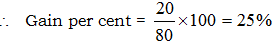QUESTION: 10

A container containing 400 litres of oil lost 8% by leakage. Oil left on the container is

Solution:

Remaining oil in container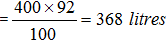QUESTION: 11

In an election, three candidates contested. The first candidate got 40% votes and the second got 36% votes. If total number of votes polled were 36000, find the number of votes got by the 3rd candidate.

Solution:

Vote percentage of third candidate
= 100 – 40 – 36 = 24%
∴ Votes got by third candidate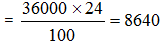QUESTION: 12

A train, 120 m long, takes 6 seconds to pass a telegraph post; the speed of train is

Solution:

Speed of train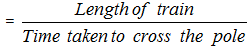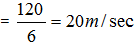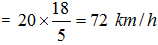QUESTION: 13

Atrain travelled at a speed of 35 km/hr for the first 10 minutes and at a speed of 20 km/hr for the next 5 minutes. The average speed of the train for the total 15 minutes is

Solution:

Distance covered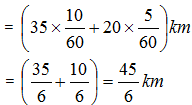Total time = 15 minutes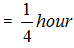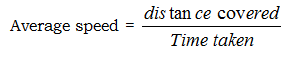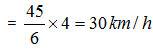QUESTION: 14

If x : y :: 2 : 3 and 2 : x : 4 : 8 the value of y is

Solution:

Here x : y :: 2 : 3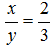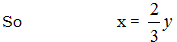2 : x :: 4 : 8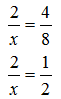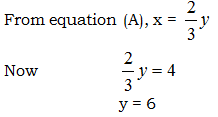QUESTION: 15

If 40% of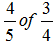of a number is 48, then what is 1% of the same number?

Solution:

Here 40% ofof number = 48
Let number = x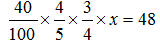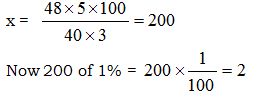QUESTION: 16

A train 50 metre long passes a platform 100 metre long in 10 sec. The speed of the train in km/hr is

Solution:

Speed of train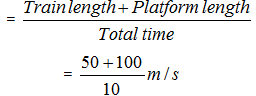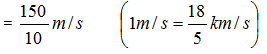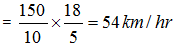QUESTION: 17

Rs. 730 were divided among A, B, C in such a way that if A gets Rs. 3, then B gets Rs. 4 and if B gets Rs. 3.50 then C gets Rs. 3. The share of B exceeds that of C by

Solution:

Given   A : B = 3 : 4
B : C = 3.50 : 3
(Here A = Rs 3, B = Rs4)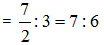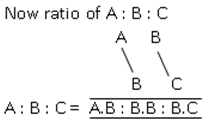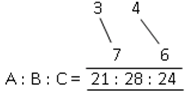Now share of B exceeds that of C by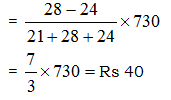QUESTION: 18

Mahesh earned a profit of 20% by selling 60 apples at the rate of Rs. 51 for 6 apples. Then the total cost, at which the apples were bought is

Solution:
QUESTION: 19

In a prep school, the average weight of 30 girls in a class among 50 students is 16 kg and that of the remaining students is 15.5 kg. What is the average weight of all the students in the class?

Solution:

Average weight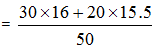QUESTION: 20

A boy found that the average of 20 numbers is 35 when he writes a number ‘61’ instead of ‘16’. The correct average of 20 numbers is

Solution:

Average of 20 numbers = 20 x 35 = 700
Here difference of a number = 61 – 16 = 45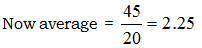Now correct average of 20 number = 35 – 2.25 = 32.75